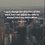# Magnets of different shapes

Hello everyone...
I am very confused..
I wanted to know that if we cut a magnet (bar magnet) diagonally into two equal halves, then what is the pole strength and magnetic moment of each piece if the magnetic moment and pole strength of the bar magnet( before cutting ) is $M$ and $m$.
I know that if we cut a bar magnet into two halves transversely or longitudinally ,the magnetic moment will be half in both the cases( how, that i also know )...
Also, i wanted to know that if we cut a magnet ( rectangular ) into magnets of different shapes( say triangle, square, rectangle etc) in different ways, then how to calculate magnetic moments of different shaped pieces... compared to the magnetic moment of initial uncut magnet..

I searched many books but I was unable to clear my doubt..

If anyone has questions of such types, then please tell me ( with a solution)...

Thank you.4 years, 10 months ago

This discussion board is a place to discuss our Daily Challenges and the math and science related to those challenges. Explanations are more than just a solution — they should explain the steps and thinking strategies that you used to obtain the solution. Comments should further the discussion of math and science.

When posting on Brilliant:

• Use the emojis to react to an explanation, whether you're congratulating a job well done , or just really confused .
• Ask specific questions about the challenge or the steps in somebody's explanation. Well-posed questions can add a lot to the discussion, but posting "I don't understand!" doesn't help anyone.
• Try to contribute something new to the discussion, whether it is an extension, generalization or other idea related to the challenge.

MarkdownAppears as
*italics* or _italics_ italics
**bold** or __bold__ bold
- bulleted- list
• bulleted
• list
1. numbered2. list
1. numbered
2. list
Note: you must add a full line of space before and after lists for them to show up correctly
paragraph 1paragraph 2

paragraph 1

paragraph 2

[example link](https://brilliant.org)example link
> This is a quote
This is a quote
    # I indented these lines
# 4 spaces, and now they show
# up as a code block.

print "hello world"
# I indented these lines
# 4 spaces, and now they show
# up as a code block.

print "hello world"
MathAppears as
Remember to wrap math in $$ ... $$ or $ ... $ to ensure proper formatting.
2 \times 3 $2 \times 3$
2^{34} $2^{34}$
a_{i-1} $a_{i-1}$
\frac{2}{3} $\frac{2}{3}$
\sqrt{2} $\sqrt{2}$
\sum_{i=1}^3 $\sum_{i=1}^3$
\sin \theta $\sin \theta$
\boxed{123} $\boxed{123}$

Sort by:

???

- 4 years, 10 months ago